## What are Perpendicular and Parallel Lines?

Practice Unlimited Questions

#### What are perpendicular lines?

When two lines meet at a right angle, the lines are called perpendicular lines.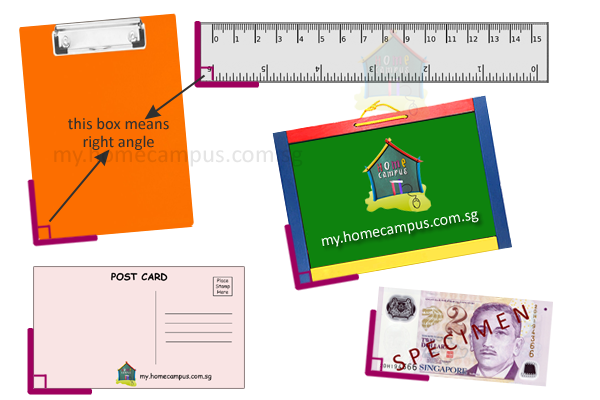On each object, the two adjacent lines marked in purple are said to be perpendicular to each other.

#### What are parallel lines?

Two lines are parallel to each other if the distance between them is always the same and they will never meet no matter how long they are drawn.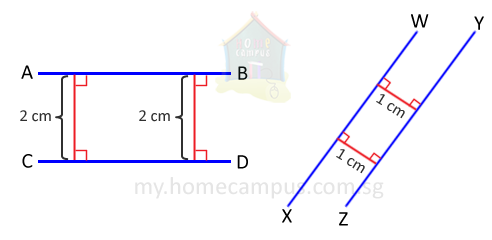Above are two examples of parallel lines.
Line AB is parallel to Line CD.
Line WX is parallel to Line YZ.

## Identifying Perpendicular Lines

#### 1. Which of the following pairs of lines are perpendicular lines?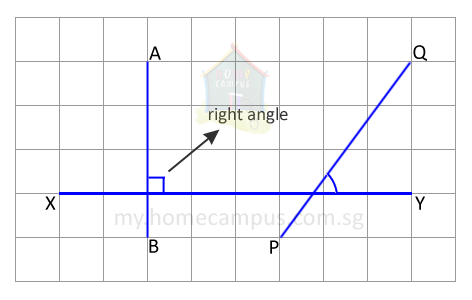AB and XY cross at a right angle.
So, the two lines are perpendicular lines.
We use the symbol ⊥ to say is perpendicular to.
In the figure, AB ⊥ XY.
Lines PQ and XY do not meet at a right angle.
So, the two lines are not perpendicular lines.

#### 2. The following figure is a rectangle.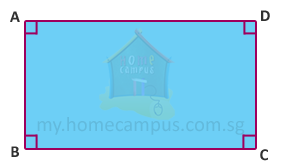Lines AB and BC are perpendicular to each other.
Can you find another pair of perpendicular lines in the figure above?

#### 3. ST is a straight line. Draw a line perpendicular to it.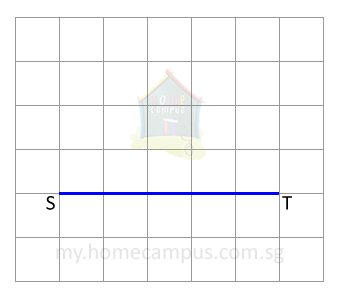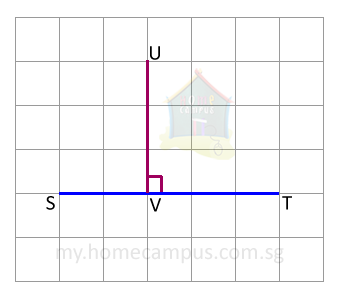The perpendicular line must lie along the grid.

#### 4. PQ is a straight line. Draw a line perpendicular to it.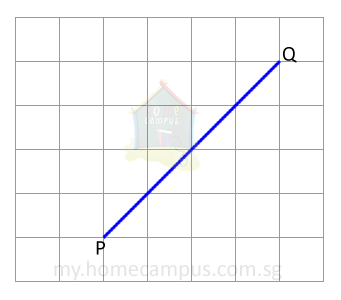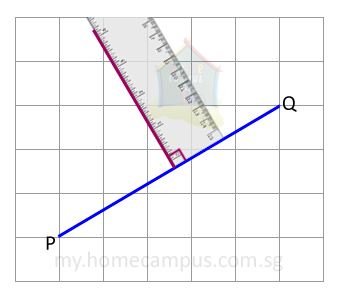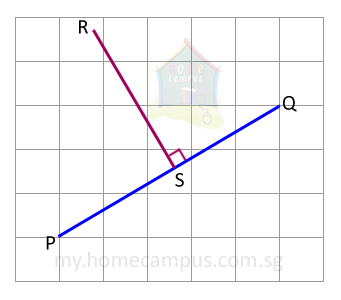## Identifying Parallel Lines

#### 5. Which of the following pairs of lines are parallel lines?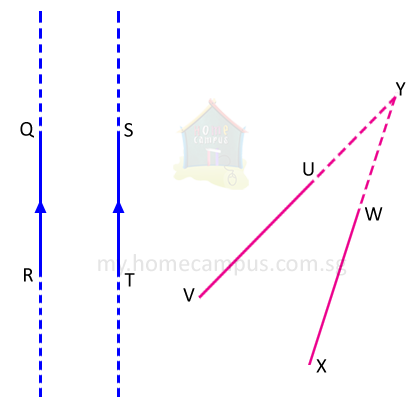Lines QR and ST do not meet each other even when they are extended.
So, the two lines are parallel lines.
We use the symbol // to say is parallel to. In the figure, QR // ST.
To show two lines are parallel to each other, we mark them by arrowheads.
Lines UV and WX meet each other when extended.
So, the two lines are not parallel lines.

#### 6. Here are some objects around us with parallel lines.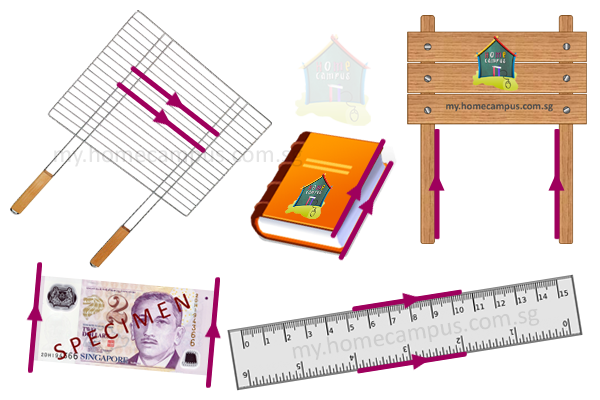To show two lines are parallel to each other, we mark them by arrowheads.

#### 7. The following figure is called a parallelogram.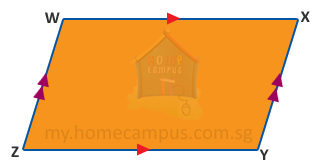Lines WX and ZY are parallel to each other.
Can you find another pair of parallel lines in the figure above?

#### 8. EF is a straight line. Draw a line parallel to it.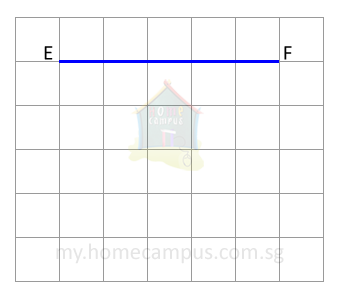The distance between the two lines must be the same always.
In other words, the number of unit squares between the lines must be the same.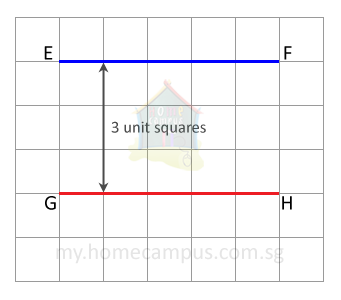The distance between EF and GH is always 3 unit squares on the grid.
So, EF // GH.

#### 9. QR is a straight line. Draw a line parallel to it.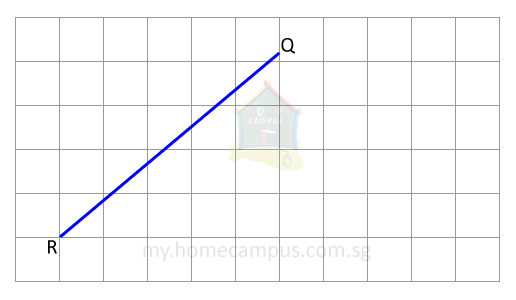Count the number of unit squares between the two lines and make sure it is always the same.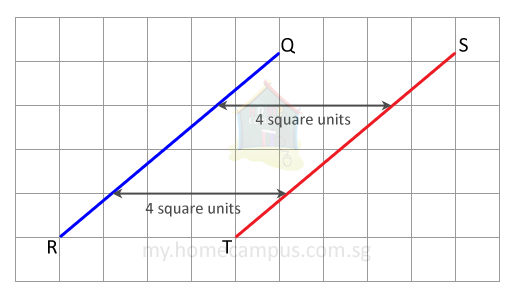The distance between QR and ST is always 4 unit squares on the grid.
So, QR // ST.